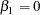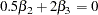The QLIM Procedure

<’label’:>

TEST <’string’:> equation [,equation...] / options ;

The TEST statement performs Wald, Lagrange multiplier, and likelihood ratio tests of linear hypotheses about the regression parameters in the preceding MODEL statement. Each equation specifies a linear hypothesis to be tested. All hypotheses in one TEST statement are tested jointly. Variable names in the equations must correspond to regressors in the preceding MODEL statement, and each name represents the coefficient of the corresponding regressor. The keyword INTERCEPT refers to the coefficient of the intercept.

The following options can be specified in the TEST statement after the slash (/):

ALL

requests Wald, Lagrange multiplier, and likelihood ratio tests.

WALD

requests the Wald test.

LM

requests the Lagrange multiplier test.

LR

requests the likelihood ratio test.

The following illustrates the use of the TEST statement:

```   proc qlim;
model y = x1 x2 x3;
test x1 = 0, x2 * .5 + 2 * x3 = 0;
test _int: test intercept = 0, x3 = 0;
run;
```

The first test investigates the joint hypothesis thatandOnly linear equality restrictions and tests are permitted in PROC QLIM. Tests expressions can be composed only of algebraic operations involving the addition symbol (+), subtraction symbol (-), and multiplication symbol (*).

The TEST statement accepts labels that are reproduced in the printed output. TEST statement can be labeled in two ways. A TEST statement can be preceded by a label followed by a colon. Alternatively, the keyword TEST can be followed by a quoted string. If both are present, PROC QLIM uses the label preceding the colon. In the event no label is present, PROC QLIM automatically labels the tests.Previous Page | Next Page | Top of Page